# Software

## Featured!The simulator is an R package for making the code one writes for simulation studies more organized and structured, which streamlines the process, saving time and making one's code easier to understand and easier to share. [website] [paper] [github] [CRAN]

## Covariance estimationggb is an R package for graph-guided banding of the covariance matrix. This generalizes the notion of a banded covariance matrix to situations where the variables lie on a known seed graph. [paper] [github]varband is an R package for learning local dependence in ordered data (such as data collected over time or along a genome). It estimates a lower triangular matrix with a variable bandwith, leading to an easily interpretable view of the extent of the local dependence in the data. [paper] [github] [CRAN] [vignette]hierband is an R package for convex banding of the covariance matrix. [paper] [CRAN] [vignette]spcov is an R package for sparse estimation of the covariance matrix. [paper] [CRAN]

## Prototype selection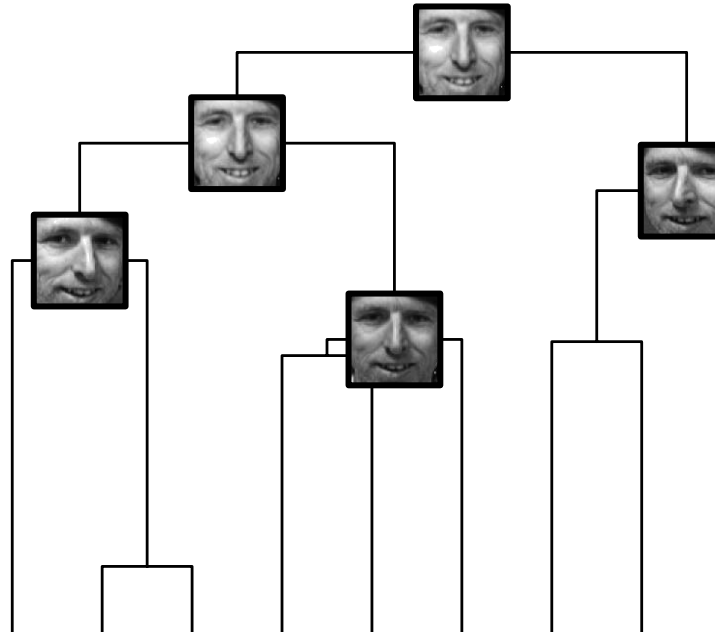protoclust is an R package for prototype clustering (i.e., minimax linkage hierarchical clustering). It creates dendrograms that have a prototypical example associated with each interior node. [paper] [CRAN]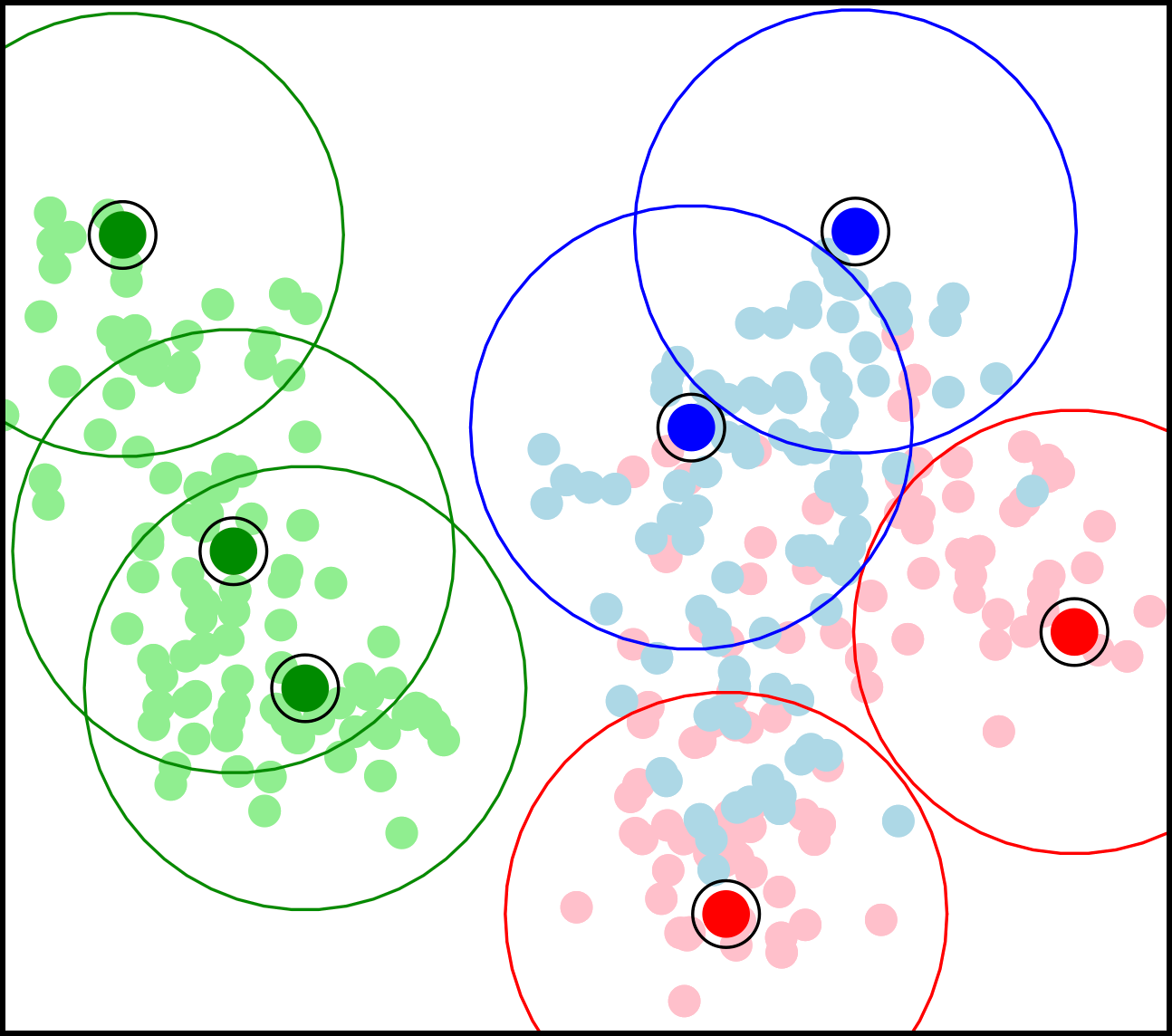protoclass is an R package for interpretable classification with prototypes. [pdf] [CRAN]

## Interaction modelinghiertest is an R package for convex hierarchical testing of interactions. [paper] [CRAN]hiernet is an R package for a lasso for hierarchical interactions. [paper] [CRAN]

## High-dimensional time series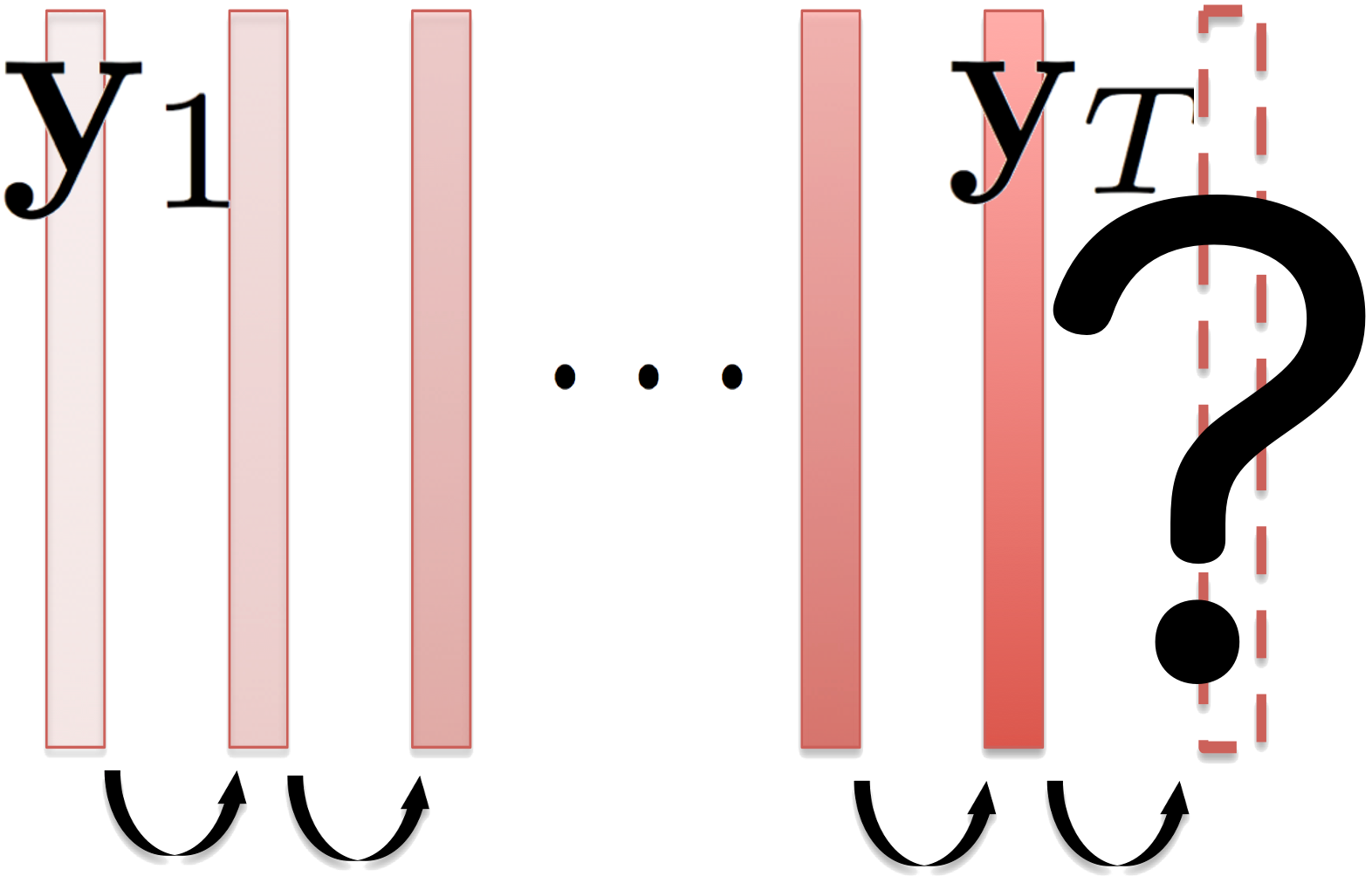BigVAR is an R package for high-dimensional time series. It implements multiple convex-regularized approaches to estimating vector autoregressive models. This includes methods for adaptively learning the lag order and for incorporating exogenous time series. [paper on HVAR] [paper on VARX-L] [CRAN] [github]

## Inferential tools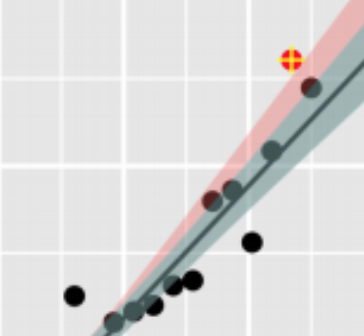outference is an R package for performing valid inference that accounts for outlier removal. [paper] [github]

## Optimization-focused software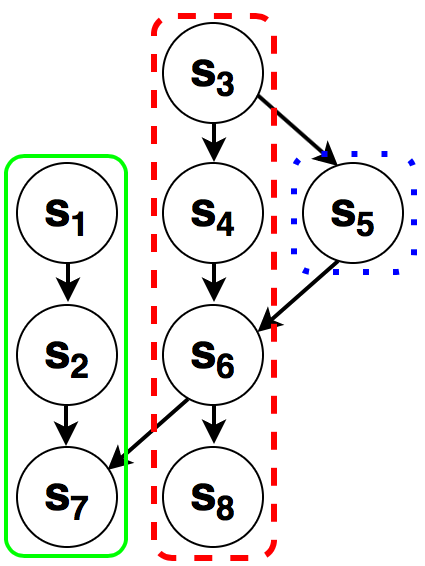hsm is an R package for hierarchical sparse modeling (HSM). This includes a novel closed-form solution to the proximal operator of the latent overlapping group lasso in the case of a directed path graph HSM structure and an efficient algorithm, which we call “path-based block coordinate descent” for more general HSM structures. [paper] [CRAN]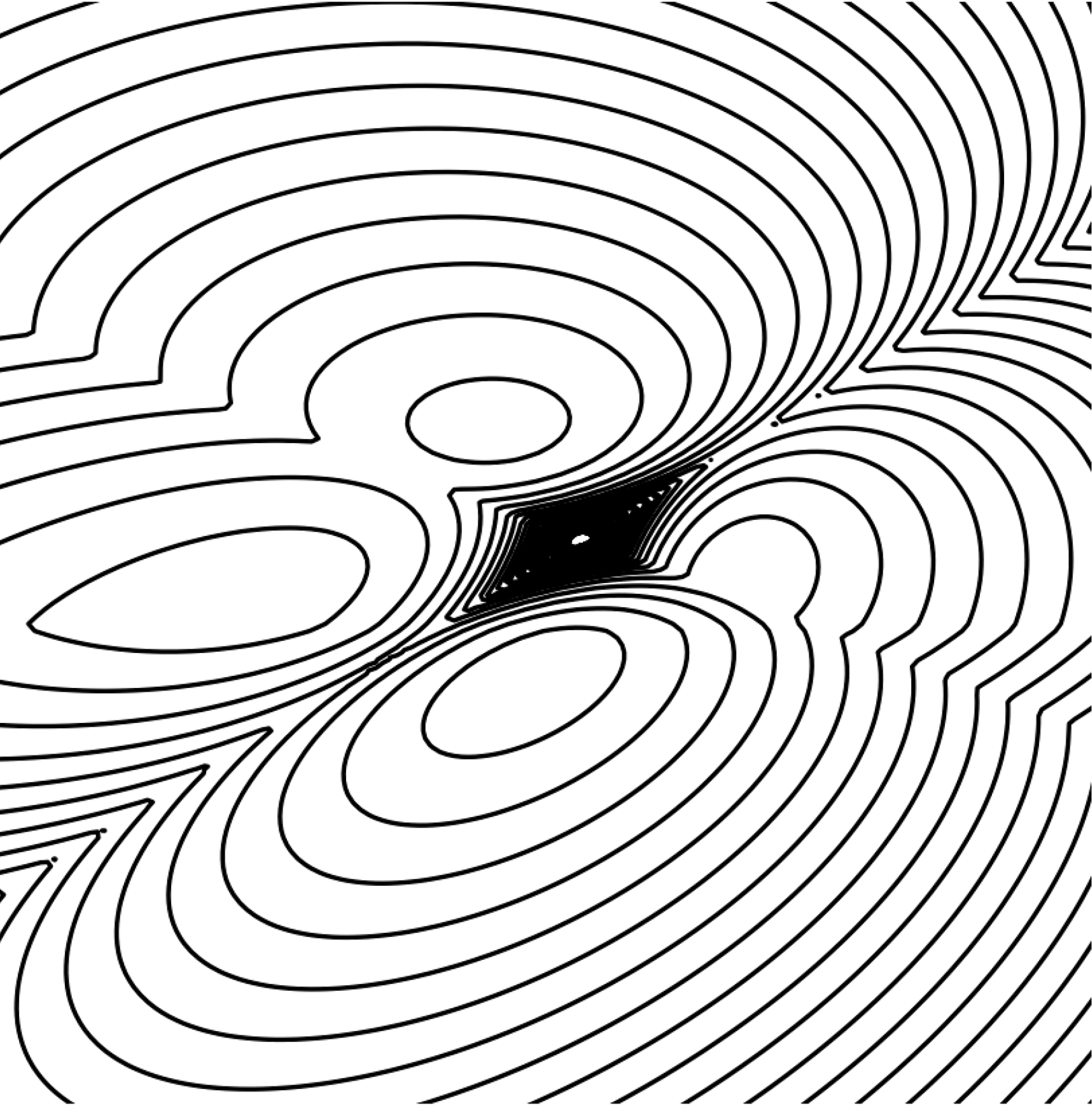TREX is Matlab code for solving the TREX, a non-convex optimization problem that arises in high-dimensional statistics. Remarkably, this code globally optimizes a non-convex optimization problem in polynomial time. [paper] [github]CVXfromR is an R package that lets you call CVX (from Matlab) within R. CVX is a tool by Michael Grant and Stephen Boyd that makes it easy to solve general convex problems, and the syntax to input such problems is simple and straightforward. [website]convexjulia is an R package that lets you call Convex.jl (from Julia) within R. Convex.jl is a package in Julia for disciplined convex programming (DCP). Similar to CVX in MATLAB, Convex.jl makes it easy to solve general convex problems (for more details, see here). [website]